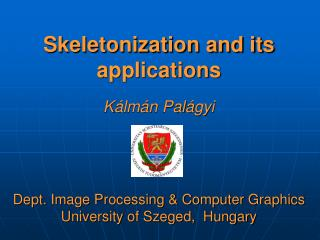# Syllabus - PowerPoint PPT PresentationDownload PresentationSyllabus

SyllabusDownload Presentation## Syllabus

- - - - - - - - - - - - - - - - - - - - - - - - - - - E N D - - - - - - - - - - - - - - - - - - - - - - - - - - -
##### Presentation Transcript

1. Skeletonization and its applicationsKálmán PalágyiDept. Image Processing & Computer Graphics University of Szeged, Hungary

2. Syllabus • Shape features • Skeleton • Skeletonization • Applications

3. Syllabus • Shape features • Skeleton • Skeletonization • Applications

4. The generic model of a modular machine vision system (G.W. Awcock, R. Thomas, 1996)

5. Feature extraction – shape representation (G.W. Awcock, R. Thomas, 1996)

6. Shape It is a fundamental concept in computer vision. It can be regarded as the basis for high-level image processing stages concentrating on scene analysis and interpretation.

7. Shape It is formed by any connected set of points. Examples of planar shapes (L.F. Costa, R. Marcondes, 2001)

8. Shape representation • to describe the boundary that surrounds an object, • to describe the region that is occupied by an object, • to apply a transform in order to represent an object in terms of the transform coefficients.

9. Contour-basedshape representation • chain-code • run-length • polygonal approximation • syntactic primitives • spline • snake / active contour • multiscale primitives (L.F. Costa, R. Marcondes, 2001)

10. Region-basedshape representation • polygon • Voronoi / Delaunay • quadtree • morphological decomposition • convex hull / deficiency • run-length • distance transform • skeleton (L.F. Costa, R. Marcondes, 2001)

11. Region-basedshape representation • polygon • Voronoi / Delaunay • quadtree • morphological decomposition • convex hull / deficiency • run-length • distance transform • skeleton (L.F. Costa, R. Marcondes, 2001)

12. Syllabus • Shape features • Skeleton • Skeletonization • Applications

13. Skeleton • result of the Medial Axis Transform: object points having at least two closest boundary points; • praire-fire analogy: the boundary is set on fire and skeleton is formed by the loci where the fire fronts meet and quench each other; • the locus of the centers of all the maximal inscribed hyper-spheres.

14. Nearest boundary pointsand inscribed hyper-spheres

15. Object = union of the inscribed hyper-spheres object boundary,maximal inscribed disks and their centers

16. Skeleton in 3D The skeleton in 3D generally contains surface patches (2D segments).

17. Skeleton →Original object

18. Skeleton →Original object

19. Skeleton →Original object

20. Skeleton →Original object

21. Skeleton →Original object

22. Skeleton →Original object

23. Skeleton →Original object

24. Skeleton →Original object

25. Skeleton →Original object

26. Skeleton →Original object

27. Skeleton →Original object

28. Uniqueness The same skeleton may belong to different elongated objects.

29. Inner and outer skeleton (inner) skeleton outer skeleton (skeleton of the negative image)

30. Stability

31. Representing the topological structure

32. Properties • represents • the general form of an object, • the topological structure of an object, and • local object symmetries. • invariant to • translation, • rotation, and • (uniform) scale change. • simplified and thin.

33. Syllabus • Shape features • Skeleton • Skeletonization • Applications

34. „Skeletonization”

35. Skeletonization … … means skeleton extraction from elongated binary objects.

36. Skeleton-like descriptors in 3D medial surface original medial lines topological kernel

37. Example of medial surface S. Svensson (SUAS, Uppsala)

38. Example of medial lines

39. Skeletal points in 2D – points in 3D centerlines

40. Example of topological kernel original image topological kernel

41. Example of topological kernel simply connected → an isolated point multiply connected →closed curve

42. Example of topological kernel

43. Skeletonization techniques • distance transform • Voronoi diagram • thinning

44. Skeletonization techniques • distance transform • Voronoi diagram • thinning

45. Distance transform Input: Binary array A containing feature elements (1’s) and non-feature elements (0’s). Output: Non-binary array B containing the distance to the closest feature element.

46. Distance transform output (non-binary) input (binary)

47. Distance transform using city-block (or 4) distance

48. Distance transform using chess-board (or 8) distance

49. Distance-based skeletonization • Border points (as feature elements) are extracted from the original binary image. • Distance transform is executed (i.e., distance map is generated). • The ridges (local extremas) are detected as skeletal points.

50. Distance-based skeletonization – step 1 Detecting border points